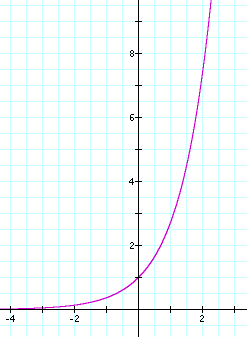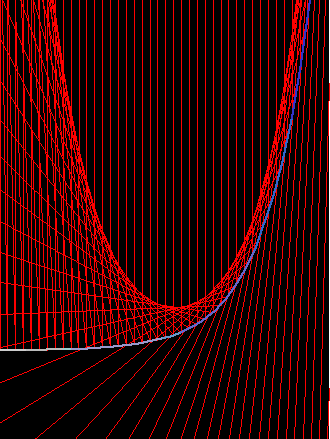# Exponential CurveThe exponential curve y==E^x

## Description

The exponential curve is defined as E^x, where E is the constant Limit[(1 + 1/x)^x, x -> ∞]

y == E^x

## Properties

### Caustic of expontial curve

The Catacaustic of the exponential curve E^x with light rays from above and parallel to the y axes is the catenary.parallel rays above the expontial curve

The exponential curve E^x has the interesting property itself. It is the only function whose derivative is itself.

## Related Web Sites

Robert Yates: Curves and Their Properties.

2006-05

If you have a question, put \$5 at patreon and message me.